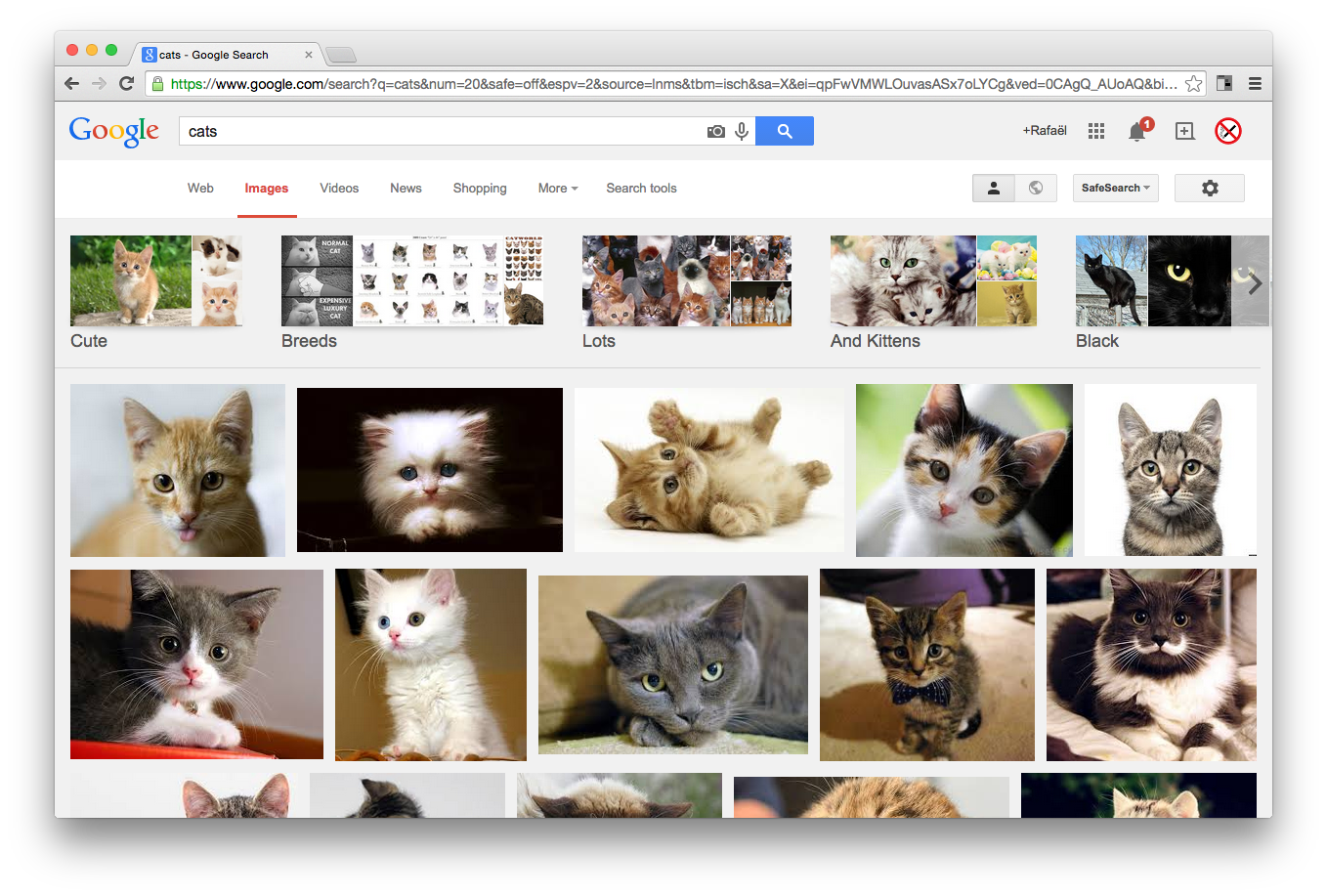# Peter’s trace the outside with booleans and functions

//trace outside
boolean mr;
boolean md;
boolean ml;
boolean mu;
int x=0;
int y=0;
int step=10;
int size=step;
void setup() {
//frameRate(2);
size(720, 480);
mr=true;
}
void draw() {
drawRect();
test();
if (mr) {
moveRight();
}
if (md) {
moveDown();
}
if (ml) {
moveLeft();
}
if (mu) {
moveUp();
}
println(mr,md,ml,mu, step);
}
void drawRect() {
fill(random(255));
rect(x, y, size, size);
}
void moveRight() {
x=x+size;
if (x>=width-step) {
mr=false;
md=true;
}
}
void moveDown() {
y=y+size;
if (y>=height-step) {
md=false;
ml=true;
}
}
void moveLeft() {
x=x-size;
if (x<0+step) {
ml=false;
mu=true;
}
}
void moveUp() {
y=y-size;
if (y<0+step+1) {
step=step+size;
mu=false;
mr=true;
}
}
void test() {
if (mr) {
md=false;
ml=false;
mu=false;
}
if (md) {
mr=false;
ml=false;
mu=false;
}
if (ml) {
mr=false;
md=false;
mu=false;
}
if (mu) {
mr=false;
md=false;
ml=false;
}
}
//

# Object Demo .pde

//Declare

Ball myBall;
Ball myBall2;
Ball myBall3;

void setup() {
size(720, 480);
smooth();
//initialize, instanciate
myBall = new Ball(500, 50);
myBall2= new Ball(100, 200);
myBall3= new Ball(200, 100);
}
//call functionality, functions of your ball
void draw() {
background(0);
myBall.run();
myBall2.run();
myBall3.run();
}

class Ball {
//global variables, place to specify data, location speed etc

float x;
float y;
float speedX=2;
float speedY=.5;

//constructor, how do you build your class, what does it need and where to initialize variables

//similiar to setup, constructor happens just once

Ball(float _x, float _y) {

x=_x;
y=_y;
}

//functions: modules, things to call separetely
void run() {
//display on the screen
display();
move();
bounce();
gravity();
}
void gravity() {
speedY+=0.2;
}

void bounce() {
if (x>=width || x<=0) {
speedX=speedX*-1;
}
if (y>=height || y<=0) {
speedY=speedY*-1;
}
}
void move() {
x+=speedX;
y+=speedY;
}
void display() {
ellipse(x, y, 20, 20);
}
}

# Object tutorial files and break assignment

Watch this and follow this video:

Using the files created from this tutorial, import the function (face/character) that we created last week as an object/class. Write a program that uses at least 3 instances of your face/character that each behave differently. Just like the 3 balls are independently moving, your face will have individual characteristics. (size, location, movement, speed, color etc)

Also, write short demo programs of your assigned functions from the processing reference page. Be prepared to demo your assigned function to the class.

Have a nice break!

p

# 3 bouncers

Bouncers b,b1,b2;
int x=0;
int y;
int b1y;

int speed=1;

void setup(){
size(720,480);
b1y = 60; y=height/2;
b=new Bouncers(0,y,50);
b1 = new Bouncers(0,b1y,75);
b2 = new Bouncers(0,150,30);

}
void draw(){
x=x+speed;
b.move(x,y); b.display();
b1.move(x,b1y); b1.display();
b2.move(x,150); b2.display();
if(x>=width||x<=0){
speed=speed*-1;
}

}
class Bouncers{
int x,y;
int size;

Bouncers(int mx,int my,int msize){
x=mx;
y=my;
size=msize;
}

void move(int mx,int my){
x=mx;
y=my;
}

void display(){
ellipse(x,y,size,size);
}

}

# MyCircles Array

MyCircles [] list = new MyCircles;

void setup(){
size(720,480);
for(int x=0; x<list.length;x++){
list[x]=new MyCircles((int)random(width),(int)random(height),random(0,200),(int)random(255));
}
}

void draw(){
background(255);
noFill();

for(int x=0;x<list.length; x++){
list[x].move((int)random(width), (int)random(height));
}
}
class MyCircles{
int x,y;
float size;
int cfill;

MyCircles(int mx, int my, float msize,int mfill){
x=mx;
y=my;
size=msize;
cfill=mfill;

}
void move(int mx, int my){
x=mx;
y=my;
display();

}
void display(){
fill(cfill);
ellipse(x,y,size,size);
ellipse(x+size,y,size,size);
}

}

# Weekend Work

#1: Draw a grid of 200 randomly colored rectangles using nested for loops.

#2:Create an array of 10 colors. Create a painting tool(think mouseX, mouseY) that utilizes / cycles through each color of your array in order, and then randomly.

Bonus:use mousePressed to turn on and off cycling of color.

float greyInc, step,
greyFill, pos;

void setup(){
size(1200,480);
greyFill = pos = 0;
noLoop();
println(“greyInc = “+greyInc);
println(“step = “+step);

}

void draw(){
int i=1;
// draw rectangle with appropriate grey fill
fill(greyFill);
rect(pos,0, step,height);

// number the rectangle + print greyscale value
fill(255,0,0);
textSize(width/100); text(i,pos,50);
textSize(width/180); text(greyFill,pos,100);

// increment the grey & position
greyFill=greyFill+greyInc;
pos = pos + step;

i++;
}
}

# push pop code

//PushMatrix and PopMatrix
//palette of 7 colors
color[]roygbiv={#FF0000,#FF9900,#FAFF00,#21FF00,#006CFF,#006CFF,#006CFF};
color[]palette=roygbiv;

int s=80;

size(720,480);
smooth();
noStroke();
background(palette);
rect(0,0,s,s);

pushMatrix(); //pushing off to the side and saving the above settings
pushStyle();
translate(230,25);
scale(2);
stroke(palette);
strokeWeight(15);
fill(palette);

rect(0,0,s,s);

popMatrix(); //goes back to what it was before the PUSHMATRIX with no translate or styles
rect(350,20,s,s);

popStyle();//pops style, stroke, fill etc, back to before the push, so there is no fill or styles or translate
rect(width-s,height-s,s,s);

# basic transform code

//transform demo
//palette of 7 colors
color[]roygbiv={#FF0000,#FF9900,#FAFF00,#21FF00,#006CFF,#006CFF,#006CFF};
color[]palette=roygbiv;

int s=80;
int d=10;

size(720,480);
smooth();
noStroke();
background(palette);

fill(palette);
rect(0,0,s,s);
fill(palette);
ellipse(0,0,d,d);

//translate origin
translate(40,90);
fill(palette);
rect(0,0,s,s);
fill(palette);
ellipse(0,0,d,d);

//change scale
translate(140,-70);
scale(2);
fill(palette);
rect(0,0,s,s);
fill(palette);
scale(.5);
ellipse(0,0,d,d);

//rotate
translate(280,10);
scale(1.33);
//rotate(PI*.25);// from 0,0 and it rotates clockwise 2pie is all the way around